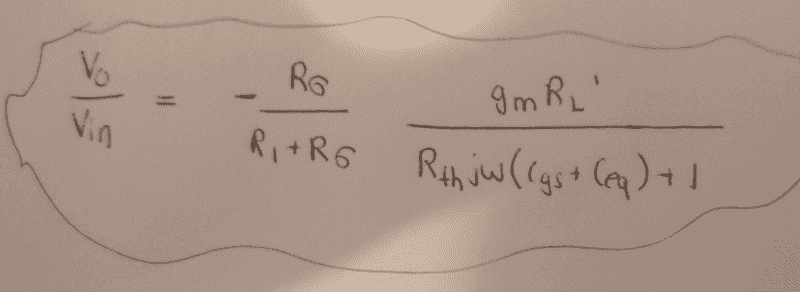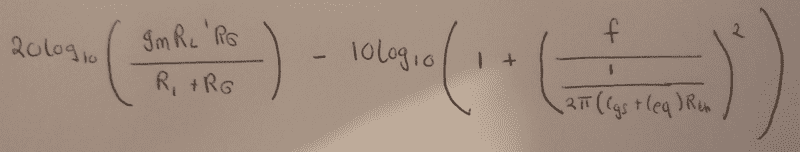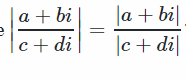# Finding the magnitude of the voltage gain in dB

## Homework Statement

Hi,
so in a MOSFET amplifier question(a common-source amplifier in particular), we are examining the effects of the parasitic capacitances of the MOSFET when included in the small-signal model. In particular, the gain of the amplifier with these capacitances.
So I derived the small-signal voltage gain including the parasitic capacitances as follows,I verified that this is correct.

Now, I'm stuck on how to find the actual magnitude of the this gain in dB. The answer just skips directly to this,I understand the first part of that, i.e thats the magnitude of the gain in dB of the top of the fraction and the following that should be the magnitude of the gain of the bottom (subtracted of course). I have no idea where second part is coming from.

## Homework EquationsdBgain = 20log10(gain)

## Answers and Replies

Charles Link
Homework Helper
Gold Member
2020 Award
First of all, they are doing a quotient of the form ## log(\frac{A}{B})=log(A)-log(B) ##. They also do a substitution ## \omega=2 \pi f ##. In addition, they used ## 20 \, log(\sqrt{C})=20 \, log(C^{1/2})=10 \, log (C) ##. (Note ## log(C^n)=n \, log(C) ##). The rest you should be able to figure out. ## \\ ## And I don't know why they put the last term in the form: ## \Big(\frac{f}{\frac{1}{(a+b)}} \Big)^2 ##. They could simply have written it as ## [ f(a+b)]^2 ##.

Last edited: